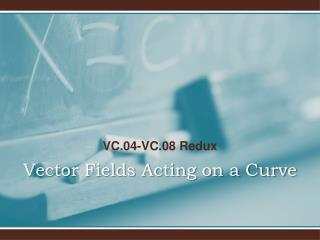# Vector Fields Acting on a Curve - PowerPoint PPT PresentationDownload PresentationVector Fields Acting on a Curve

Vector Fields Acting on a Curve
Download Presentation## Vector Fields Acting on a Curve

- - - - - - - - - - - - - - - - - - - - - - - - - - - E N D - - - - - - - - - - - - - - - - - - - - - - - - - - -
##### Presentation Transcript

1. VC.04-VC.08 Redux Vector Fields Acting on a Curve

4. VC.04: Breaking Field Vectorsinto Two Components

5. VC.04: Flow Along and Flow Across

6. VC.05: Measuring the Flow of a Vector Field ALONG a Curve These integrals are usually called line integrals or path integrals.

7. VC.05: Measuring the Flow of a Vector Field ALONG a Curve

8. VC.05: Measuring the Flow of a Vector Field Along Another Closed Curve  Counterclockwise

9. VC.05: Measuring the Flow of a Vector Field ACROSS a Curve

10. VC.05: Measuring the Flow of a Vector Field Across Another Closed Curve  Counterclockwise

12. VC.05: The Flow of a Gradient Field Along a Closed Curve

13. VC.06: The Gauss-Green Formula

14. VC.06: Measuring the Flow of a Vector Field ALONG a Closed Curve Let C be a closed curve with a counterclockwise parameterization. Then the net flow of the vector field ALONG the closed curve is measured by: Let region R be the interior of C. If the vector field has no singularities in R, then we can use Gauss-Green:

15. VC.06: Summary: The Flow of A Vector Field ALONG a Closed Curve: • Let C be a closed curve parameterized counterclockwise. Let Field(x,y) be a vector field with no singularities on the interior region R of C. Then: • This measures the net flow of the vector field ALONG the closed curve.

16. VC.06: Measuring the Flow of a Vector Field ACROSS a Closed Curve Let C be a closed curve with a counterclockwise parameterization. Then the net flow of the vector field ACROSS the closed curve is measured by: Let region R be the interior of C. If the vector field has no singularities in R, then we can use Gauss-Green:

17. VC.06: Summary: The Flow of A Vector Field ACROSS a Closed Curve: • Let C be a closed curve parameterized counterclockwise. Let Field(x,y) be a vector field with no singularities on the interior region R of C. Then: • This measures the net flow of the vector field ACROSS the closed curve.

18. VC.06: The Divergence Locates Sources and Sinks Let C be a closed curve with a counterclockwise parameterization with no singularities on the interior of the curve. Then:

19. VC.06: The Rotation Helps You Find Clockwise/Counterclockwise Swirl Let C be a closed curve with a counterclockwise parameterization with no singularities on the interior of the curve. Then:

20. VC.06: Avoiding Computation Altogether

21. VC.06: A Flow Along Measurement With a Singularity

22. VC.06: A Flow Along Measurement With a Singularity

23. VC.06: A Flow Along With Multiple Singularities? No Problem!

24. VC.06: Flow Along When rotField(x,y)=0

25. VC.06: Flow Along When divField(x,y)=0

26. VC.07: The Area Conversion Factor:

27. VC.07: The Area Conversion Factor:

28. VC.07: : Fixing Example 2

29. VC.08: 3D Integrals:

30. VC.08: A 3D-Change of Variables with an Integrand